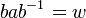# Presentation of semidirect product is disjoint union of presentations plus action by conjugation relations

(diff) ← Older revision | Latest revision (diff) | Newer revision → (diff)

## Statement

### In terms of external semidirect product

Suppose$N$ is a group and$H$ is a group acting on$N$, i.e., a homomorphism of groups$\rho:H \to \operatorname{Aut}(N)$ is specified. Suppose we are given presentations for both$N$ and$H$, and assume further that the generating sets for$N$ and$H$ have already been made disjoint, i.e., no generator letter is repeated between the two presentations.

The External semidirect product (?)$N \rtimes H$ can be given the following presentation:

• Generating set is taken as the union of generating sets for$N$ and$H$.
• Relation set is taken as the union of relation sets for$N$ and$H$ and the following action relations: for every generator$a$ of$N$ and every generator$b$ of$N$, the relation$bab^{-1} = w$ where$w$ is the word (in$N$) for the element$\rho(b) \cdot a$).